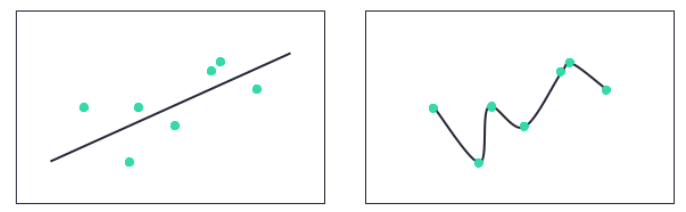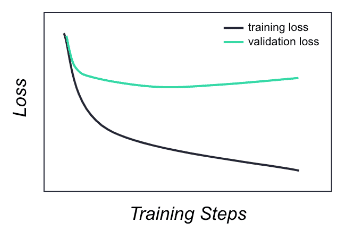Event

Meet Us at ODSC West in San Francisco from Oct 31-Nov 1

# What Is Overfitting in Machine Learning?

In supervised learning, machine learning (ML) models are trained to perform a specific predictive task by learning from information conveyed in historical data. This training set is created to be representative of real-world data, i.e., the data that the model will predict on, in order to maximize the chances that the model will behave as expected at inference time.

Sometimes, supervised models specialize too heavily on the noisy and incomplete patterns present in the training set and fail to generalize to new data. This concept is called overfitting.

This article introduces the definition of overfitting, the reasons it occurs, and how to identify and compensate for its occurrence.

## What Is Overfitting?

Model overfitting is a statistical error in supervised machine learning, whereby the trained model fits the noise in the training data rather than its actual pattern.

Let’s look at an example regression use case to better understand what overfitting is.

##Figure 1: Two example regression lines for the same data points, without overfitting (left) and with overfitting (right)

On the left, we have a linear regression model that fits the training data points without overfitting. On the right, we have a non-linear regression model that perfectly fits the same training data points.

The model on the right provides perfect performance metrics on the training set, and thus would seem to outperform the model on the left. When completing model evaluation on the test data, which is unseen at training time, we will learn that the opposite is true. The model on the right is unlikely to perform well (“generalize”) on the unseen data set, unless the prediction data fortuitously follows the overfitted line.

## Why Does Overfitting Occur?

Overfitting in supervised machine learning can occur for a variety of reasons, the most common being:

• The training set is too small: The data from which the model learns is not representative of the full spectrum of data that it needs to predict on.
• The training set is too noisy: The data from which the model learns contains incorrect or missing information, limiting the model’s ability to learn a generalizable pattern.
• The model is too complex: The model learns too precisely the patterns in the training set, including irrelevant information.
• The model’s training regime is not tuned for the data: The parameter selection for the algorithm determines its complexity, and an incorrect selection results in a model definition or training regime that leads to overfitting.

We should also note that while overfitting is not a term associated with unsupervised ML due to the absence of a correct label, it is still possible to set up these algorithms in such a way that leads to a loss of generalization. An example is deriving n clusters on a data set of size n.

## How To Identify Overfitting

Model overfitting is commonly identified in two ways. The first is by comparing performance on the training set and test set. Optimal performance on the training set with largely lower performance on the test set is a sign of overfitting. The second method of identifying overfitting is by analyzing validation metrics, most typically the loss curve.

##Figure 2: Plot showing training and validation losses for an example overfitting model

When a model is overfitting, the validation metric—in the example plots above, the validation loss—stops improving, and even begins deteriorating after some training steps, while the training metric keeps improving. Also, overfitted models often show low bias and high variance.

## How To Compensate for Overfitting

When a machine learning model overfits, it can lose its value as a predictive tool. This is why we typically want to compensate for overfitting.

Common modeling techniques to consider are:

• Early stopping: The model training is paused when n training steps have been completed without performance improvement, where n is a user-defined parameter typically recommended to be set as 10% of the total training iterations.
• Regularization: The complexity of the model is reduced by applying a penalty to the loss function which minimizes—or even cancels out—the contribution of less important features and weights.
• Ensembling: The predictions of more than one model are combined in order to generate a more accurate prediction.
• Cross-validation: The model is trained iteratively on different train and test splits of the original data in order to ensure a more generalized performance.

Data can also be treated directly to alleviate overfitting via data augmentation, further data collection, and thorough feature selection. The latter aims to reduce noise in the data, while the two former techniques aim to increase the training set size and variety.

For both modeling and data techniques, it is important to be aware that over-applying the techniques to compensate for overfitting can lead to the opposite effect, i.e., underfitting (the model does not learn enough information from the training set.) It is recommended to apply changes iteratively, and to keep track of the model’s performance. An experiment tracking system such as MLRun can provide the support needed to run experiments robustly, collaboratively, and efficiently.